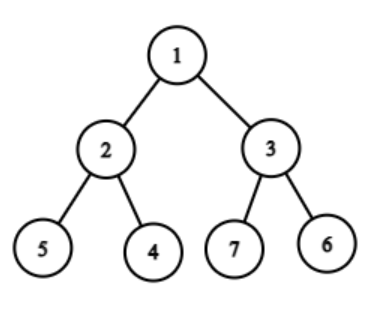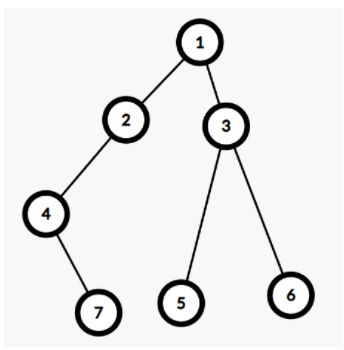Posted: 2 Dec, 2020
Difficulty: Moderate

## PROBLEM STATEMENT

#### Any node whose left and right child are null is called a leaf node in a binary tree.

##### Example:
``````Consider the given binary tree:
````````````For the given tree the leaf nodes are 5, 4, 7, 6. All these nodes are on the same level. So the output will be True.
``````
##### Input format :
``````The first line contains a single integer T representing the number of test cases.

The first line of each test case contains elements in the level order form(refer to the example below) (separated by space). If any node does not have a left or right child, take -1 in its place.
``````
##### Example:
``````Elements are in the level order form. The input consists of values of nodes separated by a single space in a single line. In case a node is null, we take -1 in its place.

For example, the input for the tree depicted in the below image would be :
1
2 3
4 -1 5 6
-1 7 -1 -1 -1 -1
-1 -1
````````````Explanation :
Level 1 :
The root node of the tree is 1

Level 2 :
Left child of 1 = 2
Right child of 1 = 3

Level 3 :
Left child of 2 = 4
Right child of 2 = null (-1)
Left child of 3 = 5
Right child of 3 = 6

Level 4 :
Left child of 4 = null (-1)
Right child of 4 = 7
Left child of 5 = null (-1)
Right child of 5 = null (-1)
Left child of 6 = null (-1)
Right child of 6 = null (-1)

Level 5 :
Left child of 7 = null (-1)
Right child of 7 = null (-1)

The first not-null node (of the previous level) is treated as the parent of the first two nodes of the current level. The second not-null node (of the previous level) is treated as the parent node for the next two nodes of the current level and so on.

The input ends when all nodes at the last level are null (-1).

Note: The above format was just to provide clarity on how the input is formed for a given tree.

The sequence will be put together in a single line separated by a single space. Hence, for the above-depicted tree, the input will be given as:

1 2 3 4 -1 5 6 -1 7 -1 -1 -1 -1 -1 -1
``````
##### Output format :
``````For each test case, print "True" if all the leaf nodes are at the same level otherwise print "False” (without quotes).

The output of each test case should be printed in a separate line.
``````
##### Note:
``````You are not required to print anything, it has already been taken care of. Just implement the function.
``````
##### Constraints:
``````1 <= T <= 100
1 <= N <= 3000

Time Limit: 1 sec
``````Approach 1

We can run a breadth-first search (BFS) on the binary tree and find out the levels of all the leaves in the binary tree. If the levels of all the leaves in the binary tree are equal, we return True else we return False.

Algorithm:-

1. Run a breadth-first search (BFS) from node 1.
2. Make an empty queue and push node 1 into the queue and update its level as 0.
1. Pop the first element in the queue. (Lets its level be ‘X’)
2. Update the levels of its right child (if present) and left child (if present) as ‘X’+1.
3. Push the right child (if present) and left child (if present) into the queue.
4. If both right and left child is not present then the node is a leaf and hence store its level in a separate array.
5. Repeat the algorithm till the length of the queue is greater than 0.
3. Iterate through the array ‘ARR’ (from 1 to ‘N’ where ‘N’ is the length of the array) in which all the levels of the leaf nodes are stored (say the iterator be i).
4. Check whether ‘ARR[i]’ is equal to ‘ARR’. If no, return False.

4. If the elements in the array ‘ARR’ are equal, return True.